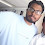# Pharma Engineering

For Engineer By Engineer

• ## Thursday, 30 June 2016

Hello Everyone.....!! Back after many days, with a Basic post, this involves only basic which many of the guyz have forgotten after entering Pharma Industries, I don't mean that Pharma Industry is the main reason but i just mean that while involving a high degree of calculations Engineers will prefer to use appropriate figures rather than accurate figures, but we need to keep one thing in mind that whenever we keep enlarging our vision we need to remember the basic things, that's the reason i have taken this topic here.

Basically while trying to calculate the density one should be aware of what does a density mean exactly and what units does it holds, as well as how to calculate it for a mixture when two or more solvents was involved from individual densities.

What is Density ?

The Basic definition that everyone knows is Mass/Volume, and it will hold the units as Kg/Cu.m in SI units, and gm/cc in CGS units.

How to determine the density of a solvent practically ?

As this site particularly deals with Pharma Industry i've mentioned directly SOLVENT in the query without mentioning as liquid, please don't bother about that. The simple way to determine the density of a solvent in real life is to take some known volume in a bottle or a container and weigh it, calculate the net weight of the solvent in the bottle/container, then divide the weight with the known volume, then the derived figure will be Density, but please take care of the units that you consider, i'm not responsible for any mistake that was done by you by considering wrong units.

How to calculate the density of solvent mixture from individual densities ?

This is somewhat a difficult task for some guyz because there lies 3 equations hitting up our mind while we think to calculate but within those three equations there lies only one correct equations, now i'll give you those three equations below,

Equation 1 :

Density of Binary Mixture = ( Mass fraction of Component 1  *  Density of component 1 ) + ( Mass fraction of component 2 * Density of component 2 ),

Simply, D = ( X1 * D1 ) + ( X2 * D2 ),

Equation 2 :

Density of Binary Mixture = 1 /  ( ( Mass fraction of Component 1  *  Density of component 1 ) + ( Mass fraction of component 2 * Density of component 2 ) ),

Simply, D = 1 /  ( ( X1 * D1 ) + ( X2 * D2 ) ),

Equation 3 :

Density of Binary Mixture = 1 / ( ( Mass Fraction of Component 1 / Density of Component 1) +  (Mass Fraction of component 2 / Density of Component 2 ) ),

Simply, D = 100 / ( ( X1 /D1 ) + ( X2 / D2 )).

And Apart from these three equations some guyzz will do some funny calculations as Mixture Density = ( Component 1 Density + Component 2 Density ) / 2, this is very cheap calculation, so my dear engineers learn the correct way don't do calculation like this one.

Getting into the matter seriously, Out of the three equations those were mentioned above the third one is a perfect one, you may have a doubt that why did i said that as perfect instead of Correct, because based on some special cases the Equations 1 & 2 may be applicable but not to all cases.

So the Above mentioned Equation 3 is perfect one while you want to calculate from Mass fractions, and there is some other way of determining the Mixture density that is based on volume fractions or volume percentage.

i.e., Density of Binary Mixture = ( ( Volume % of solvent 1 * Density of solvent 1) + (Volume % of Solvent 2 * Density of solvent 2 ) ) ,

Simply, D = ( ( ( V1 / V ) * D1) + ( ( V2 / V ) * D2 ) ).

So, i think now you got what is the exact procedure to calculate the Density of a Mixture, if you have any queries please feel free to contact us,

If you understand what i delivered above, Say Cheer's.

All Valuable Comments are appreciated. Thanks :)Hi! I am Ajay Kumar Kalva, Currently serving as the CEO of this site, a tech geek by passion, and a chemical process engineer by profession, i'm interested in writing articles regarding technology, hacking and pharma technology.

1.2.Sir,
Can you please tell me how you have taken 100 in equation -3.
regards,

1.Dear Sarfaraz,

That is for 100%, so i've taken 100 over there.

Regards,
AJAY K

3.Can I use equation 3 for ternary solution mixture?
i.e.,
D= 100/((X1/D1)+(X2/D2)+(X3+D3))
Is above equation valid for ternary solution mixture?

Regards
Kumar Shivesh
Department of Chemical Engineering and Technology
Indian Institute of Technology ( Banaras Hindu University) Varanasi Uttar Pradesh

1.hi amanulla from AP, guntur. your articles are much much useful for every chemical engineer

4.Thank you so much. I forgot the basics while dealing with Gladstone-Dale equation...😅

## This Blog is protected by DMCA.comHi! I am Ajay Kumar Kalva, owner of this site, a tech geek by passion, and a chemical process engineer by profession, i'm interested in writing articles regarding technology, hacking and pharma technology.